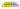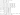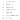# Simplification and Approximation

Hello all,

As you all know that simplification is a crucial topic of quantitative aptitude. In this post, we will discuss some of the important methods for solving simplification problems from which minimum five questions may ask in almost every competitive exams i.e. IBPS PO, IBPS CLERK, SBI PO, SBI CLECK, SSC CGL, SSC CHSL ETC.

Questions based on simplification is used to check the understanding and fast calculating capability of candidates. Just you have to follow the basic principle i.e.  The BODMAS concept:-

BODMAS it stands for –

1. The order of solving bracket:-
2. a) Bar Bracket (–):- when an expression contains Bracket, before applying BODMAS rule, we simplify the expression under Bracket.
3. b) Circular bracket ( ).
4. c) Curly bracket { }.
5. d) Square bracket [ ].
6. Of- Stand for power like square, cube.
7. D – Stand for the division.
8. M- Stand for multiplication.
10. S – Stand for subtraction.

Note: – While solving the question you must go left to right.Tips for fast calculation of simplification Problems:-

• By heat squares of numbers up to 30 and cubes of numbers up to 15.
• It is easy to solve in Fractions as compared with decimals. So, instead of 0.67 solve in fraction 2/3.
• Remember tables up to 20.
• Use unit place concept.
• Remember Algebraic identities.
• Learn to approximation and rounding off numbers.

Approximation:-

Unlike simplification problems where we can find exact output by simplifying the expression, the approximate value is required in approximation problems, there is no need to find the exact answer of the question, but it is close to the exact answer.

Types of questions:-

In banking exam, the questions based on simplification are of following types:

1. Simple and based on Bodmas rule.
2. Questions involving fractions, decimals, and percentage based on BODMAS rule.
3. Questions involving algebraic identities which are as follows:-4. Questions based on surd and idicies.5. Question involving approximation.

So, let us discuss a few examples based on this with their solution.

Direction: (1-3) In Question-based on algebraic identities What will come in the place of ?.

• (3.28-1.72)2 +4×3.28×1.72=?
1. a) 21 b) 25 c) 24 d) 20 e) None of these.

2)  450 ×450-150×150 =?

450+150

a)200 b) 100 c) 240 d) 300 e) None of these.

3)     (1000)9 ÷ 1024=?

1. a) 10 b)100 c)1000 d)None of these.

(3.28-1.72)2 +4×3.28×1.72=?

From the above statement, identify that the statement is based on the algebraic identity, i.e. (a-b)2+4ab that can be written as (a+b)2.

Therefore,

a=3.28 b=1.72

(3.28+1.72)2= (5)2 = 25.

450 ×450-150×150     =?

450+150

From the above statement, identify that the statement is based on the algebraic identity, i.e. a2 +b2= (a+b) (a-b).

(450+150)2= (450+150) (450-150) = 300

450+150

From the above statement, identify that the statement is based on surd and indices.

(1000)9 ÷ 1024=?

= (103)9 ÷102 4  [(am) n= am×n]

= 1027÷1024       [am ÷ an=am-n]

= 10 27-24

= 10 3

=1000

Directions: (1to10) What value will come in the place of question mark (?) in the questions as follows?

1. 318 × 5160 ÷ 43 % of 2400 = 46 × 990 ÷ 15 + ? –2400
2. a) 1026 b) 954 c) 1288 d) 1342 e) None of these.
3. 45 % of 600 – 18 % of 350 =? – 62 – 252
4. a) 728 b) 756 c) 894 d) 912 e) None of these
5. 2516 ×? + 16 × 11 = 2526 + 5198
6. a) 6 b) 3 c) 8 d) 10 e) None of these.
7. 1/7 x 1/5 x 4/9 x 3150 + 40% of 7800 =? – 540
8. a) 3700 b) 5600 c) 4400 d) 3200 e) None of these
9. 1/19 x 7638 + 1/26 x 2080 =? x 241
10. a) 10 b) 5c) 5.2 d) 2 e) None of these
11. 343.55 + 533.43 –4444.35 + 3353.54 =? – 333.33
12. a) 115.3 b) 113.6 c) 119.5 d) 101.4 e) None of these
13. 80% of 24000 – 16% of 7200 =? – 15% of 2000
14. a) 12360 b) 18348 c) 20650 d) 16408 e) None of these
15. ∛35937 +√5184 ÷ 2x– 4/5 of 65 + 52 = 42
16. a) 1 b) 5 c) 10 d) 14 e) None of these
17. 6 (3/4) + 4 (3/8) – 6 (¼) =? – 5 (½)
18. a) 11 (3/8) b) 12 (5/8) c) 10 (3/8) d) 14 (1/3) e) None of these.
19. (16)2 × (28)2 =?

(64)

1. a) 59 b) 56 c) 78 d 49 e) None of these.

Solutions:-

318 × 5160 ÷ [(43/100) × 2400] = 46 × 990 ÷ 15 +? –2400

318 × (5160/1032) = 46× (990/15) +? – 2400

1590 = 3036 +? – 2400

1590 + 2400 – 3036 =?

? = 954

45 % of 600 – 18 % of 350 = x – 62 – 252

(45/100)600 – (18/100) ×350 + 62 + 625 =?

? = 270 – 63 + 62 + 625

? = 894

2516 × x + 16 × 11 = 2526 + 5198

2516 × x + 176 = 7724

2516 × x = 7548

x = 7548/2516 = 3

1/7 × 1/5 × 4/9 ×3150 + 40% of 7800 =? – 540

= > 40 + (40/100) ×7800 + 540 =?

= > 40 + 3120 + 540 =?

= > 3700 =?

(1/19) x 7638 + (1/26) x 2080 =? × 241

= > 402 + 80 =? × 241

= > 482/241 =?

= > 2 =?

343.55 + 533.43 – 4444.35 + 3353.54 =? – 333.33

= > – 213.83 =? – 333.33

= > 333.33 – 213.83 =?

= > 119.5 =?

80% of 24000 – 16% of 7200 =? – 15% of 2000

= > (80/100) x 24000 – (16/100) x 7200 =? –

(15/100) x 2000

= > 19200 – 1152 =? – 300

= > 19200 – 1152 + 300 =?

= > 18348 =?

∛35937 + √5184 ÷ 2x – 4/5 of 65 + 52 = 42

33 + (72÷ 2x) – (4/5) ×65 + 25 = 42

(72÷ 2x) = 42 – 33 – 25 + 52

(72÷ 2x) = 36

2x = 72÷ 36 = 2

2x = 21

x = 1

6 (3/4) + 4 (3/8) – 6 (¼) + 5 (½) = x

(6 + 4 – 6 + 5) (3/4 + 3/8 – ¼ + ½) = x

X = 9 (11/8)

X = 10 (3/8)

(16)2 × (28)2   =?

(64)

=16 ×16×28×28 =   7×7 =49

64×64

Approximation

Direction (1-18): What value will come in the place of question mark (?) in the questions as follows?

1. 998% of 680.06+130.001% of 449.998+20.01%of 125.089=?
2. a) 1222 b) 1322 c) 1422 d) 1522 e) None of these.
3. √6560*4.007+119.99*1.998-50.001% of 479.908=?
4. a) 348 b) 324 c) 368 d) 372 e) 396
5. 99+111.011+37.01*2.999+44.99% of 180=?
6. a) None of these b) 484 c) 424 d) 414 e) 464
7. 01*1.998+450.01% of 890-1999.99+24.998*1.11=?
8. a) 2390 b) 2480 c) 2220 d) 2440 e) None of these.
9. ?*5+444.99*5.99+899.906+9.999=4579.99
10. a) 200 b) 300 c) 280 d) 320 e) 400
11. 99% of 789.907 * 15.08 + 111.999 ÷ 4.034 -45% of 399.998 =?
12. a) 2220 b) 2290 c) 2190 d) 1320 e) None of these.
13. 998 * 3.98 + 15.998 * 2.076 + 90.078 * 7.996 =?
14. a) 782 b) 982 c) 882 d) 892 e) 872
15. √3970 ÷998 + 5.098 * 268.99 + 433.908 =?
16. a) 1800 b) 2000 c) 1700 d) 2100 e) None of these.
17. 23 * 12.998 + 34.03 ÷ 16.999 + 24.01 ÷ √575.902=?
18. a) 302 b) 312 c) 322 d) 332 e) None of these.
19. 99 ÷ 5.07 + √6240.99 * 1.998 – 111.11 =?
20. a) 67 b) 42 c) 65 d) 55 e) None of these.
21. 989÷7.05-4.998*17.96+14.05*3.99-12.09=?
22. a) 54 b) 60 c) 48 d) 42 e) 64
23. (12.05)2-(8.99)2+ (13.99)2-(17.08)2=?
24. a) -80 b) -43 c) -30 d) -15 e) None of these.
25. √99.99*20.05%of 319.998+√3599.99+? =800.067
26. a) 120 b) 100 c) 110 d) 130 e) None of these.
27. 08*√196.45-9.99% of 2000.08-25.99*8.99=?
28. a) 504 b) 510 c) 520 d) None of these e) 514
29. 99*3.04+80.03% of 40.06-12.977*1.907+?=45.06*3.009
30. 20 b) 28 c) 18 d) 24 e) None of these.
31.   856 =? + 428 – (315 ÷ 4 × 8.74)
32. a) None of these.b) 2160 c) 2780 d) 2520 e) 1140
33. 713.41 – 263.78 ÷ (21.78 % of 201) =?
34. a) 825 b) None of these c) 636 d) 707 e) 913
35. 26 % of 349.75 – 32 % of 599.27 =? – 157.94 –(27.21)2
36. a) 820 b) 568 c) 786 d) 634 e) None of these.

Solutions:

Approximation:-

89.998% of 680.06+130.001% of 449.998+20.01% of

125.089=?

90/100 * 680 + 130/100 * 450 + 20/100 * 125 =?

612+585+25=?

1222=?

√6560*4.007+119.99*1.998-50.001% of 479.908=?

81*4+120*2-240=?

324=?

110.99+111.011+37.01*2.999+44.99% of 180=?

111+111+111+81=?

414=?

180.01*1.998+450.01% of 890-1999.99+24.998*1.11=?

360+4005-2000+25=?

2390=?

?*5+444.99*5.99+899.906+9.999=4579.99

?*5+445*6+900+10=4580

?*5=1000

?=200

19.99% of 789.907 * 15.08 + 111.999 ÷ 4.034 – 45% of

399.998 =?

2370 + 28 – 180 =?

2218 =? = 2220

34.998 * 3.98 + 15.998 * 2.076 + 90.078 * 7.996 =?

35 * 4 + 16 * 2 + 90 * 8 =?

892 =?

√3970 ÷ 2.998 + 5.098 * 268.99 + 433.908 =?

21 + 1345 + 434 =?

1800 =?

23 * 12.998 + 34.03 ÷ 16.999 + 24.01 ÷ √575.902 =?

299 + 2 + 1 =?

302 =?

99.99 ÷ 5.07 + √6240.99 * 1.998 – 111.11 =?

20 + 158 – 111 =?

67 =?

699.989÷7.05-4.998*17.96+14.05*3.99-12.09=?

700 ÷ 7 – 5*18+14*4-12 =?

100-90+56-12=?

54=?

(12.05)2-(8.99)2+ (13.99)2-(17.08)2=?

144-81+196-289=?

-30=?

√99.99*20.05% of 319.998+√3599.99+? =800.067

√100 * 20/100 * 320 + √3600 +? = 800

10*64+60+? =800

?=100

67.08*√196.45-9.99% of 2000.08-25.99*8.99=?

67 * √196 – 10/100 * 2000 – 26*9 =?

67*14-200-234=?

504=?

34.99*3.04+80.03% of 40.06-12.977*1.907+?

=45.06*3.009

35*3 + 80/100 * 40 – 13 *2 +? = 45*3

105 + 32 – 26 +? = 135

?=24

856 = ? + 428 – (316 ÷ 4 × 9)

856 = ?+ 428 – [(79) ×9]

856 = ?+ 428 – 71

? = 856 – 428 + 711 = 1139

?= 1140

713.41 – 263.78 ÷ (21.78 % of 201) =?

713 – 264 ÷ [(22/100)*200] =?

? = 713 – 264/44

= 713 – 6 = 707

26 % of 350 – 32 % of 600 =?– 158 – 272

(26/100)*350 – (32/100)*600 =? – 158 – 729

91 – 192 + 158 + 729 =?

? = 786